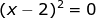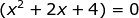## Friday, November 28, 2014

### Sketching the Graph of a Polynomial, 8

Category: Analytic Geometry

"Published in Vacaville, California, USA"

Sketch the graph of a polynomial:

Solution:

Consider the given equation above

Did you notice that the given equation can be factored by grouping? Let's factor the above equation as follows

and then it is factorable by the difference of two cubes as follows

If we set y = 0, then we can solve for the value of x at each factors which are the x-intercepts.

For, the value of x is

For, the value of x is

Since the roots of the second factor are imaginary numbers, then we cannot accept those values. Because of this, the only x-intercept is 2.

If we set x = 0, the y-intercept of the given equation is

Since we now the x-intercept, then we can sketch the location or direction of a curve as follows

If x < 0, then y = (-)²(+) = (+)
If 0 < x < 2, then y = (-)²(+) = (+)
If x > 2, then y = (+)²(+) = (+)

Here's the graph of a polynomial:Photo by Math Principles in Everyday Life